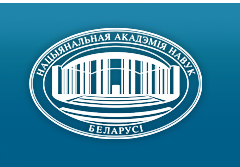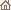Home News About Academy Academy Activities Academy Membership Organizations Publications Conferences Contacts Site Map

# Proc. of the Natl. Academy of Sciences of Belarus, Ser. Phys.-Math. Sci., 2004, No.3/ Publications / Scientific Journals

Proc. of the Natl. Academy of Sciences of Belarus, Ser. Phys.-Math. Sci., 2004, No.3Proceedings of the National Academy of Sciences of Belarus (Vestsi Natsiyanalnai Akademii Navuk Belarusi) SERIES OF PHYSICAL-MATHEMATICAL SCIENCESPublished by The Institute of Mathematics of NASB, Minsk, Republic of Belarus

### Number 3, 2004## CONTENTS

### MATHEMATICS

Kilbas A. A., Marzan S. A.
Approximate method for solution of a class of differential equations of fractional order. pp. 5--9

Summary: The Cauchy-type problem for a nonlinear differential equation of fractional order with the Riemann -- Liouville fractional derivative in a weighted space of Holderian functions is investigated. The method based on an approximation of the solution by summator polynomial operators, is applied to obtain an approximate solution of this problem. The estimate for an error of the approximate solution is established and an example is given.

Rybachenko I. V.
Approximation of functions with integrable derivatives by interpolational and rational operators. pp. 10--16

Summary: The estimation of deviation of special rational interpolating operator in uniform metrics for functions, which have fractional derivative from Lp, was found. This estimation has the order which better than the best polynomial approximation.

Gromak E. V.
Generalized Yh-transform in Lv,r-spaces. pp. 17--21

Summary: Generalized integral transform involving Bessel function of the second kind in the kernel is studied in the weighted spaces of r-summable functions. Mapping properties such as the boundedness, the representations and the range of the considered transform are given, and the inversion formulae are established.

Monastyrnyi P. I., Shvakel A. I.
Date-driven orthogonal sweep method for net equations with strongly varying coefficients. pp. 22--28

Summary: The construction and justification of the date-driven orthogonal method allowing to extend class of the solving net boundary problems with strongly varying coefficients and to provide the stability of the method under more weak than traditional restrictions are given.

Sabuts A. V.
Criterions of existence of even integral positive definite quadratic forms. II. pp. 29--34

Summary: It is established, that n-ary even integral positive definite quadratic forms with invariant factors 1 ... , 1, q1, q1q2 exist if only if the values n, q1 and q2 satisfy one from follow conditions. First, n, q1 is odd, q2 is even, and q1 or 2q2 is not square. Second, n = ± l(mod8), q1 = (2k - 1)2, q2 = 2s2, k = 1, 2,. .., s = 1,2, .. . Third, n = 0(mod4), q1 is odd, q2 = ±l(mod4), and q1 or q2 is not square. Fourth, n = 0(mod 8), q1 = (2k - 1)2, q2 = (2s - 1)2, k = 1, 2, ... , s = 1, 2, . . . Fifth, n = 0(mod4), q1 is even, q2 = ±l(mod4). Sixth, n = 2(mod4), q1 is arbitrary, q2 = - 1(mod4). Seventh, n, q1, q2 is even.

Kharin Yu. S., Maevskiy V. V.
Local-median method of regression forecasting under outliers. pp. 35--42

Summary: The local-median method of forecasting under the regression model with outliers is analyzed. The breakdown point is evaluated, the asymptotic expansion for the probability distribution function and the risk of the local-median forecast are given. Comparison of the robustness of the least square method and the local-median method is made.

Yudin M. D.
On indispensable conditions of convergence of distributions of the sums of dependent random vectors with unrestricted dispersion. pp. 43--46.

Summary: There are indispensable conditions of convergence of distributions of sums of dependent random vectors in case of unrestricted dispersion. The canonical representation of limiting distributions under the formula extending the formula Levy -- Khinchin is indicated.

Vilanski Y. V., Lepin V. V.
Information leakage in mappings with different length images. pp. 47--53

Summary: The cryptographic strength of mappings f : {0, l}n ® Umi=r{0, 1}i, is investigated. The exact expressions and estimations for the expected value of the information leakage of randomly selected mappings are presented.

Melnikov O. I., Vopnyarskiy A. V.
Realization of hypergraphs by trees of minimal diameter. pp. 54--57

Summary: The algorithm which allow to construct the realization of hypergraphs by trees of minimal diameter with complexity O(min(|VH|,|EH|)|EH| åei ÎHE |ei| proposed.

### PHYSICS

Kovalev A. A, Zhdanovich S. N.
Features of the relief-phase holograms development on photother-moplastic materials by laser method. pp. 58--62

Summary: It is shown that the developing of the relief-phase holograms on photothermoplastic materials with pulsed solid-state Nd:YAG-laser it is necessary to use intermediate temperature level situated between initial and recording temperature. The dependence of the hologram diffraction efficiency on laser radiation energy density is established.

Kats P. B., Feranchuk I. D.
Regularization of the total scattering cross-section at the Coulomb potential. pp. 63--69

Summary: Angular distribution of the particles scattered by the Coulomb potential is considered on the basis of non-asymptotic representation of the exact solution of the Schrodinger equation. It is shown that in this case the differential scattering cross-section has no singularity at zero angle. The total and transport cross-sections are calculated and proved to be finite values even for non-screening Coulomb potential. The results are important for transport processes in plasma and impurity semiconductors.

Minkevich A. A., Feranchuk I. D.
Modification of Debye -- Waller factor at large scattering angles. pp. 70--75

Summary: The modified expression for Debye -- Waller factor is derived for scattering of x-rays from rough surfaces and interfaces. For the large values of scattering vector, the non-Gaussian behavior of this factor is predicted. This effect is caused by natural limitation of roughness fluctuation amplitude and is found can be essential for interpretation of x-ray reflectivity and high-order x-ray diffraction data. Some experimental x-ray reflectivity measurements are interpreted using the expression derived.

Sevruk B. B.
Tuning performance of the sum-frequency irradiation generation in nonlinear KTP crystal. pp. 76--80

Summary: The collinear three-frequency interaction of light waves in the biaxial KTP crystal is considered. Using analytical expressions for the angular dependence of eigen mode refractive indices and the Sellmeier dispersion formulas, the wavelengths satisfied phase-matching conditions, are found. The tuning curves are constructed for the generation of sum-frequency irradiation in the X1X2 and X2X3 planes at different directions of the wave normal vector. The angular dependencies of pump and second harmonic wavelengths, as well as effective nonlinear coefficients are calculated.

Chorny A. D., Babenko V. A., Hierro J.
Gradient magnitude of turbulent passive scalar field fluctuations and its one-point PDF. pp. 81--86

Summary: The closed equation of scalar gradient magnitude PDF for turbulent mixing problem is obtained. The closure is built on base of assumption about Gaussian conditional joint PDF of components of velocity gradient and scalar gradient tensors. Numerical integration of the model equation is carried out and the obtained results are compared with direct numerical simulation of turbulent scalar field.

Karolik A. S., Luhvich A. A.
Finding the contribution of dislocations to the thermoelectric power of copper and silver. pp. 87--90

Summary: On measurements the thermoelectric power induced by tensile plastic deformation the change of thermoelectric power per unit of dislocation density DSd/Nd is fined for copper (99.998%) and silver (99.99%) at temperature close to the room one. The given measurements are the first attempt of defining the value DSd/Nd in these metals and are in the rather good agreement with the earlier performed calculations.

Makovetskii G. I., Galias A. I., Demidenko O. F.
Structure, density and magnetic susceptibility of Mn1-xFexSe (x £ 0.45) and Mn1-xCoxSe (x £ 0.1) solid solutions. pp. 91--93.

Summary: By solid state reaction method the Mn1-xFexSe (x £ 0.45) and Mn1-xCoxSe (x £ 0.1) solid solutions were synthesized. All samples have the cubic lattice like MnSe. The lattice parameter a decreases when the concentration of iron and cobalt increases. The density of all samples is calculated from X-ray measurements and measured experimentally. The magnetic susceptibility for all solid solutions is determined in temperature interval 77 -- 1000 K. The Neel temperatures of all investigated samples are about 180 K.

### INFORMATICS

Benediktovich V. I.
Noncrossing k-factors in geometric graphs. I. pp. 94--99.

Summary: It is proved that deciding the existence of a noncrossing 1-factor in orthogonal geometric graph G with r(G) ³ 1, the existence of a noncrossing 3-factor in 4-regular geometric graph G with r(G) ³ 1 and the existence of a noncrossing 2-factor in geometric graph G with r(G) ³ 1, where r(G) is the index of intersection of geometric graph G, are NP-complete.

Adutskevich E. V., Likhoded N. A.
Necessary and sufficient conditions for preservation of dependencies when paralleling of algorithms. pp. 100--105

Summary: Necessary and sufficient conditions for preservation of dependences when paralleling of algorithms are obtained. In many cases the approach suggested allows us to get less constraints which do not depend on external variables then the known ones.

Lis A. V., Sidorenko L. A., Goshko D A.
The infinite time ruin probability in the model of collective risk with Levy processes. pp. 106--111

Summary: The infinite time ruin probability is considered. The non-classical feature where the income premium process is stochastic process with independent increments as well as the aggregate claim. Under these conditions the theorem about infinite time ruin probability is proved, the equation for an adjustment coefficient is obtained. Special cases of stochastic processes with independent increments are considered. The expressions for an adjustment coefficients are obtained.

### SHORT NOTES

Zhestkov S. V., Zabreiko P. P.
One way of constructing of invariant Banach space and global theorems of solvability Cauchy problem. pp. 112--114

Summary: The way of constructing of invariant Banach space for matrix systems of ordinary differential equations is suggested. The global theorems of solvability Cauchy problem on the base of principles Banach — Cattioppoli and Kantorovich are obtained.

Sinkevich D. V.
On the calculation of the estimate of the initial state vector of the regular Markov chain. pp. 115--117

Summary: Some statistical results about estimation of the initial state vector on the basis of the aggregated information are given.

Krushevski A. E., Kondratyuk V. F.
On the problem of equilibrium of the elastic rectangular with mixed boundary conditions. pp. 118--120

Summary: Original solution of one problem of elasticity theory with Galiorkin method is presented.

### ABSTRACTS OF DEPOSITED PAPERS

Shmuratko A. S.
Degree of convergence in the central limit theorem for the weakly dependent random variables. p. 121

### SCIENTISTS OF BELARUS

Vitaliy Antonovich Tolkachev (On the occasion of the 70th birthday). pp. 122--123
Click
here to read this article in Russian (Win 1251)

Pavel Andreevich Apanasevich (On the occasion of the 75th birthday). pp. 124--125
Click here to read this article in Russian (Win 1251)/ Publications / Scientific Journals / Back to Top of Page

Designed and maintained by Dr. Nikolai N. Kostyukovich. Last updated: February 15, 2005
Created with assistance of Dr. Ignatii I. Korsak
Copyright © 2004, 2005 The National Academy of Sciences of Belarus
Copyright © 2004 The Institute of Mathematics of NASB